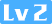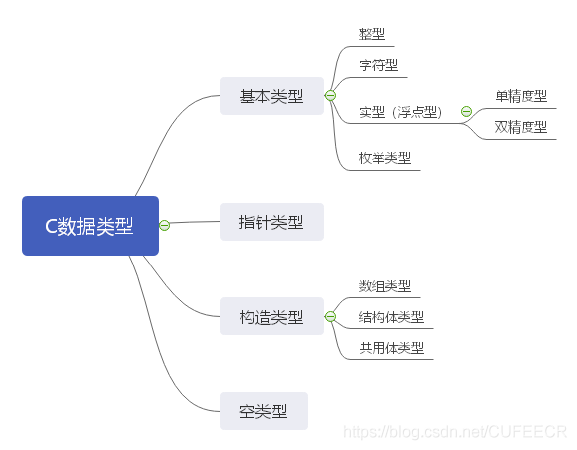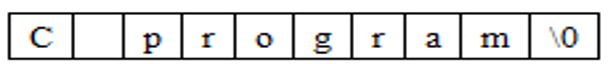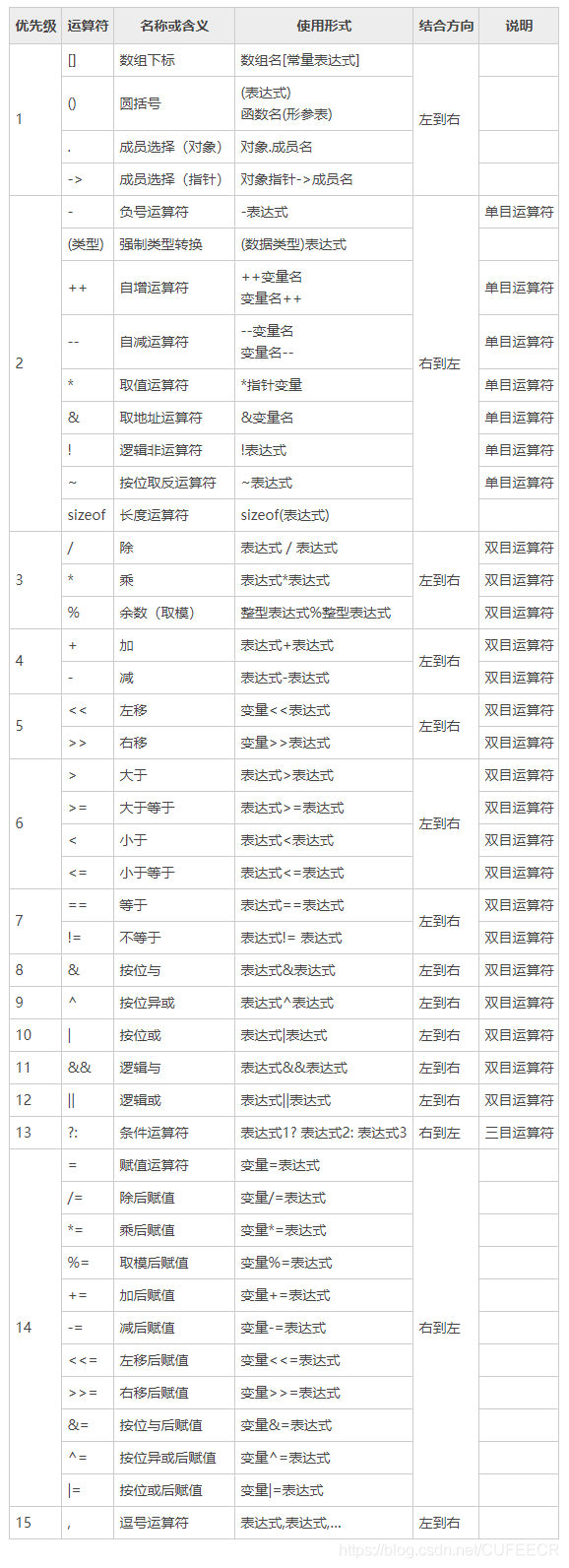C语言入门系列之2.数据类型、运算符和表达式CuterCorley373 0 0

# 一、数据类型

C语言常见数据类型如下：## 1.数据类型

• 基本数据类型 基本数据类型最主要的特点是，其值不可以再分解为其他类型。 也可以说，基本数据类型是自我说明的。
• 构造数据类型 构造数据类型是根据已定义的一个或多个数据类型用构造的方法来定义的。 也就是说，一个构造类型的值可以分解成若干个“成员”或“元素”。每个“成员”都是一个基本数据类型或一个构造类型。 在C语言中，构造类型有以下几种：
• 数组
• 结构体
• 共用体（联合）类型
• 指针类型 指针是一种特殊的、且具有重要作用的数据类型，其值用来表示某个变量在内存储器中的地址。 虽然指针变量的取值类似于整型量，但这是两个类型完全不同的量，因此不能混为一谈。
• 空类型 在调用函数值时，通常应向调用者返回一个函数值，这个返回的函数值是具有一定的数据类型的，应在函数定义及函数说明中给以说明。 例如在上一节例题中给出的max函数定义中，函数头为int max(int a, int b);，其中int类型说明符即表示该函数的返回值为整型量。 但是，也有一类函数，调用后并不需要向调用者返回函数值，这种函数可以定义为“空类型” ，其类型说明符为void。

## 2.常量和变量

### 常量和符号变量

#define 标识符 常量

#include <stdio.h>
#define PRICE 30

void main()
{
int num, total;
num = 10;
total = num * PRICE;
printf("total=%d", total);
}

total=300

• 含义清楚；
• 能做到”一改全改“。

### 变量

int k = 3;

## 3.整型数据

### 整型常量的表示方法

• 十进制整常数 十进制整常数没有前缀，其数码为0-9。 以下各数是合法的十进制整常数： 237、-568、65535、1627等。
• 八进制整常数 八进制整常数必须以0开头，即以0作为八进制数的前缀，数码取值为0-7。 八进制数通常是无符号数。 以下各数是合法的八进制数： 015(十进制为13)、0101(十进制为65)、0177777(十进制为65535)。
• 十六进制整常数 十六进制整常数的前缀为0X或0x，其数码取值为0-9、A-F或a-f。 以下各数是合法的十六进制整常数： 0X2A(十进制为42)、0XA0 (十进制为160)、0xFFFF (十进制为65535)。

### 整型变量

类型说明符  变量名标识符, 变量名标识符, ...;

int a,b,c;      // a,b,c为整型变量
long x,y;       // x,y为长整型变量
unsigned p,q;   // p,q为无符号整型变量

• 允许在一个类型说明符后，定义多个相同类型的变量，各变量名之间用“,”号间隔，类型说明符与变量名之间至少用一个空格间隔。
• 最后一个变量名之后必须以“;”号结尾。
• 变量定义必须放在变量使用之前。 一般放在函数体的开头部分。

• 正数的补码与原码相同；

• 负数的补码 将该数的绝对值的二进制形式按位取反再加1。 举例说明--求-10的补码：

10的原码： 00001010 取反： 11110101 再加1，得到-10的补码： 11110110

可以得到，最高位是符号位，1表示负数，0表示整数。

• 基本型 类型说明符为int，在内存中占4个字节。
• 短整型 类型说明符为short intshort，在内存中占2个字节。
• 长整型 类型说明符为long intlong，在内存中占4个字节。
• 无符号型 类型说明符为unsigned

#include <stdio.h>

void main()
{
printf("%d\n", sizeof(int));
printf("%d\n", sizeof(short));
printf("%d\n", sizeof(long));
printf("%d\n", sizeof(unsigned));
}

4
2
4
4

#include <stdio.h>

void main()
{
int a, b, c, d;
unsigned u;
a = 5;
b = -15;
u = 12;
c = a + u;
d = b + u;
printf("a+u=%d, b+u=%d\n", c, d);
}

a+u=17, b+u=-3

#include <stdio.h>

void main()
{
short int a, b;
a = 32767;
b = a + 1;
printf("%d, %d", a, b);
}

32767, -32768

#include <stdio.h>

void main()
{
long x, y;
int a, b, c, d;
x = 5;
y = 6;
a = 7;
b = 8;
c = x + a;
d = y + b;
printf("c=x+a=%d, d=y+b=%d", c, d);
}

c=x+a=12, d=y+b=14

## 4.实型数据

### Ⅰ.实型常量的表示方法

• 十进制小数形式 由数码0-9和小数点组成，必须有小数点。 例如0.0、25.0、5.789、0.13、5.0、300.、-267.8230等均为合法的实数。 标准C允许浮点数使用后缀，后缀为 f或F 即表示该数为浮点数，如 356f 和 356. 是等价的。

• 指数形式 由十进制数、阶码标志“e”或“E”和阶码（只能为整数，可以带符号）组成。 其一般形式为：

  aEn（a为十进制数，n为十进制整数）

例如：

2.1E5 (等于2.1105) 3.7E-2 (等于3.710-2) 0.5E7 (等于0.5107) -2.8E-2 (等于-2.810-2)

以下不是合法的实数：

345 (无小数点) E7 (阶码标志E之前无数字) -5 (无阶码标志) 53.-E3 (负号位置不对) 2.7E (无阶码)

### Ⅱ.实型变量

#### 实型数据在内存中的存放形式

• 小数部分占的位（bit）数愈多，数的有效数字愈多，精度愈高;
• 指数部分占的位数愈多，则能表示的数值范围愈大。

#### 实型变量的分类

• 单精度（float型）
• 双精度（double型）
• 长双精度（long double型）

float 32(4) 6-7 -2^128^ - 2^128^
double 64(8) 15-16 -2^1024^ - 2^1024^
long double 128(16) 18-19 -2^16384^ - 2^16384^

#### 实型数据的舍入误差

#include <stdio.h>

int main()
{
float a, b;
a = 123456.789e5;
b = a + 20;
printf("%f\n", a);
printf("%f\n", b);
return 0;
}

12345678848.000000
12345678848.000000

（1）1.0 / 3 * 3等于多少？ （2）3 / 2呢？ （3）1 / 3 * 3呢？

#include <stdio.h>

int main()
{
printf("%f\n", 1.0 / 3 * 3);
printf("%f\n", 3 / 2);
printf("%f\n", 1 / 3 * 3);
return 0;
}

1.000000
0.000000
0.000000

## 5.字符型数据

### 字符常量

• 字符常量只能用单引号括起来，不能用双引号或其它括号。
• 字符常量只能是单个字符，不能是字符串。
• 字符可以是字符集中任意字符，但数字被定义为字符型之后就不能参与数值运算。 如'5'和5是不同的，'5'是字符常量，不能参与运算。

### 字符变量

char a, b;

### 转义字符

#include <stdio.h>

int main()
{
int a, b, c;
a = 5, b = 6, c = 7;
printf(" ab c\tde\rf\n");
printf("hijk\tL\bM\n");
return 0;
}

fab c   de
hijk    M

a ='x';
b = '7';

#include <stdio.h>

int main()
{
char a, b;
a = 120;
b = 121;
printf("%c, %c\n", a, b);
printf("%d, %d\n", a, b);
return 0;
}

x, y
120, 121

#include <stdio.h>

int main()
{
char a, b;
a = 'x';
b = 'y';
printf("%c, %c\n", a, b);
printf("%d, %d\n", a, b);
return 0;
}

#include <stdio.h>

int main()
{
char a, b;
a = 'a';
b = 'b';
a = a - 32;
b = b - 32;
printf("%c, %c\n%d, %d\n", a, b, a, b);

return 0;
}

A, B
65, 66

char a = 33int a = 33的比较：

#include <stdio.h>

int main()
{
char a;
int b;
a = 33;
b = 33;
printf("%c, %c\n%d, %d\n", a, b, a, b);

return 0;
}

!, !
33, 33

### 字符串常量

• 字符常量由单引号括起来，字符串常量由双引号括起来。
• 字符常量只能是单个字符，占八位，字符串常量则可以含一个或多个字符。
• 可以把一个字符常量赋值给一个字符变量，但不能把一个字符串常量赋值给一个字符变量。 例如：可以char a = 'a';，但不能char a = "a";
• 字符常量占一个字节的内存空间，字符串常量占的内存字节数等于字符串中字节数加1，增加的一个字节中存放字符 \0 (ASCII码为0)，这是字符串结束的标志。 例如，字符串C program在内存中所占的字节示意如下：• 'a'在内存中占一个字节；
• "a"在内存中占二个字节。

## 6.变量赋初值

类型说明符 变量1 = 值1, 变量2 = 值2, ……;

int a=3;
int b, c=5;
float x=3.2, y=3f, z=0.75;
char ch1='K', ch2='P';

## 7.变量类型转换

### 自动类型转换

• 若参与运算量的类型不同，则先转换成同一类型，然后进行运算。
• 转换按数据长度增加的方向进行，以保证精度不降低。如int型和long型运算时，先把int量转成long型后再进行运算，来保证损失较低、精度较高。
• 所有的浮点运算都是以双精度进行的，即使仅含float单精度量运算的表达式，也要先转换成double型，再作运算。
• char型和short型参与运算时，必须先转换成int型
• 在赋值运算中，赋值号两边量的数据类型不同时，赋值号右边量的类型将转换为左边量的类型。如果右边量的数据类型长度大于左边时，将丢失一部分数据，这样会降低精度，丢失的部分按四舍五入向前舍入。

#include <stdio.h>

int main()
{
float PI = 3.14159;
int s, r = 5;
s = r * r * PI;
printf("s=%d\n", s);

return 0;
}

s=78

#include <stdio.h>

int main()
{
float s, PI = 3.14159;
int r = 5;
s = r * r * PI;
printf("s=%f\n", s);

return 0;
}

s=78.539749

### 强制类型转换

(类型说明符)  (表达式)

(float) a      // 把a转换为实型
(int)(x+y)     // 把x+y的结果转换为整型

• 类型说明符和表达式都必须加括号(单个变量可以不加括号)，如把(int)(x+y)写成(int)x+y则成了把x转换成int型之后再与y相加了。
• 无论是强制转换或是自动转换，都只是为了本次运算的需要而对变量的数据长度进行的临时性转换，而不改变数据说明时对该变量定义的类型

#include <stdio.h>

int main()
{
float f = 3.14159;
printf("(int)f=%d, f=%f\n", (int)f, f);

return 0;
}

(int)f=3, f=3.141590

# 二、运算符和表达式

## 1.基本算术运算符

• 加法运算符+ 加法运算符为双目运算符，即应有两个量参与加法运算，如a+b、4+8等。具有右结合性

• 减法运算符- 减法运算符为双目运算符，但“-”也可作负值运算符，此时为单目运算，如-x, -5等具有左结合性

• 乘法运算符* 双目运算，具有左结合性

• 除法运算符/ 双目运算，具有左结合性。 参与运算量均为整型时，结果也为整型，舍去小数；如果运算量中有一个是实型，则结果为双精度实型。

• 取余运算符% 双目运算，当运算符%的操作数（被除数和除数）均为整数时，结果为取余。 例如，16%5的结果为两数相除的余数1。 当运算符%的操作数中有一个或两个浮点数时，会产生语法错误。 例如8%2.5存在语法错误。

举例如下：

  #include <stdio.h>

int main()
{
printf("\n%d, %d\n", 20 / 7, -20 / 7);
printf("%f, %f\n", 20.0 / 7, -20 / 7.0);
printf("%d\n", 100 % 3);

return 0;
}

打印：


2, -2
2.857143, -2.857143
1


## 2.运算符的优先级和结合性

• 运算符的优先级 C语言中，运算符的运算优先级共分为15级，1级最高，15级最低。 在表达式中，优先级较高的先于优先级较低的进行运算，而在一个运算量两侧的运算符优先级相同时，则按运算符的结合性所规定的结合方向处理。
• 运算符的结合性 C语言中各运算符的结合性分为两种，即左结合性(自左至右)和右结合性(自右至左)。 例如算术运算符的结合性是自左至右，即先左后右。如有表达式x-y+z则y应先与“-”号结合，执行x-y运算，然后再执行+z的运算。这种自左至右的结合方向就称为“左结合性”。 而自右至左的结合方向称为“右结合性”。最典型的右结合性运算符是赋值运算符，如x=y=z,由于“=”的右结合性，应先执行y=z再执行x=(y=z)运算。 C语言运算符中有不少为右结合性，应注意区别，以避免理解错误。

C语言中运算符的优先级和结合性如下：## 3.高级运算符

### 自增、自减运算符

• 自增1运算符记为“++”，其功能是使变量的值自增1；
• 自减1运算符记为“--”，其功能是使变量值自减1。

• ++i i自增1后再参与其它运算。
• --i i自减1后再参与其它运算。
• i++ i参与运算后，i的值再自增1。
• i-- i参与运算后，i的值再自减1。

#include <stdio.h>

int main()
{
int i = 8;
printf("%d\n", ++i);
printf("%d\n", --i);
printf("%d\n", i++);
printf("%d\n", i--);
printf("%d\n", -i++);
printf("%d\n", -i--);
printf("%d\n", i);

return 0;
}

9
8
8
9
-8
-9
8

#include <stdio.h>

int main()
{
int i = 5, j = 5, p ,q;
p = (i++) + (i++) + (i++);
q = (++j) + (++j) + (++j);
printf("%d, %d, %d, %d\n", p, q, i, j);
i = 5, j = 5;
p = i++ + i++ + i++;
q = ++j + ++j + ++j;
printf("%d, %d, %d, %d\n", p, q, i, j);

return 0;
}

18, 22, 8, 8
18, 22, 8, 8

### 赋值运算符和赋值表达式

变量 = 表达式

x = a + b
w = sin(a) + sin(b)
y = i + j

• 实型赋予整型，舍去小数部分。
• 整型赋予实型，数值不变，但将以浮点形式存放，即增加小数部分(小数部分的值为0)。
• 字符型赋予整型，由于字符型为1个字节，而整型为4个字节，故将字符的ASCII码值放到整型量的低8位中，其余高位为0。
• 整型赋予字符型，只把低8位赋予字符量。

#include <stdio.h>

int main()
{
int a, b = 322;
float x, y = 8.88;
char c1 = 'k', c2;
a = y;
printf("%d\n", a);
x = b;
a = c1;
c2 = b;
printf("%f, %d, %c", x, a, c2);

return 0;
}

8
322.000000, 107, B

a+=5      // 等价于a=a+5
x*=y+7    // 等价于x=x*(y+7)
r%=p      // 等价于r=r%p

### 逗号运算符和逗号表达式

#include <stdio.h>

int main()
{
int a = 2, b = 4, c = 6, x, y;
y = (b + c,(x = a + b));    // 逗号运算符
printf("y=%d, x=%d", y, x);

return 0;
}

y=6, x=6

• 逗号表达式一般形式中的表达式1和表达式2也可以是逗号表达式。 例如表达式1，(表达式2，表达式3)形成了嵌套情形，因此可以把逗号表达式扩展为表达式1，表达式2，…表达式n，整个逗号表达式的值等于表达式n的值。
• 程序中使用逗号表达式，通常是要分别求逗号表达式内各表达式的值，并不一定要求整个逗号表达式的值。 并不是在所有出现逗号的地方都组成逗号表达式，如在变量说明中和函数参数表中逗号只是用作各变量之间的间隔符。

### 相关推荐

C++概述

C++ 基本语法
C 程序可以定义为对象的集合，这些对象通过调用彼此的方法进行交互。现在让我们简要地看一下什么是类、对象，方法、即时变量。 对象 对象具有状态和行为。例如：一只狗的状态 颜色、名称、品种，行为 摇动、叫唤、吃。对象是类的实例。 类 类可以定义为描述对象行为/状态的模板/蓝图。 方法 从基本上说，一个方法表示一种行为。一个类可以包含多个
【C 陷阱与缺陷 学习笔记】（一）词法陷阱

C/C 是比其他语言难些，但差距不大。以往很多人说 C/C 难，那是旧时代的产物。那时操作系统（例如 DOS）还没有如今这么强大的功能，像界面设计和底层设计都要靠应用程序来实现。由于 C 的高效率，高端设计大都用 C 语言和汇编语言来写，程序搞得很复杂，初学者有望尘莫及的感觉。C 的简捷表达法比 Fortran, Cobol, Pascal 和 BASIC
Swift与Objective-C混合编程之Swift与Objective-C API映射

c++11 实现单例模式
C11出来后,里面新增加了好多好用的功能 下面的单例就是使用了C11中的标准库中的mutex和unique_prt 进行内存管理的. 此单例模式不用担心内存的释放问题 pragma once include <memory include <mutex template <class T class Singleton { public: ty
C语言_练习题（一）

C语言32个关键字auto 声明自动变量short 声明短整型变量或函数int 声明整型变量或函数long 声明长整型变量或函数float 声明浮点型变量或函数double 声明双精度变量或函数char 声明字符型变量或函数struct 声明结构体变量或函数union 声明共用数据类型enum 声明枚举类型typedef 用以给数据类型取别名co
C语言入门系列之1.C语言概述和上机运行简单C程序

C语言入门系列之2.数据类型、运算符和表达式

C语言基础习题50例（一）1-5

C语言基础习题50例（二）6-10

C语言基础习题50例（三）11-15

HelloWorld开发者社区 - 开发者专属的技术社区